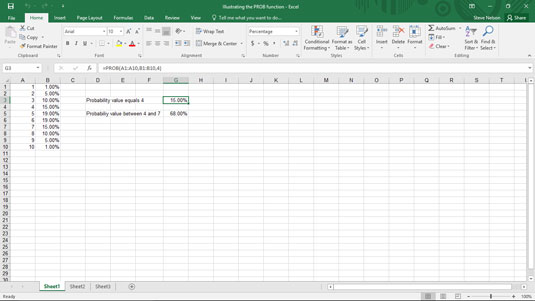##### Excel 2019 All-in-One For DummiesThe PROB function in Excel uses a set of values and associated probabilities to calculate the probability that a variable equals some specified value or that a variable falls with a range of specified values. The function uses the syntax

`=PROB(x_range,prob_range,lower_limit,[upper_limit])`

where x_range equals the worksheet range that holds your values and prob_range holds the worksheet range that specifies the probabilities for the values from x_range. To calculate the probability that a variable equals a specified value, enter that value using the lower_limit argument. To calculate the probability that a variable falls within a range, enter the bounds of that range using the lower_limit and upper_limit arguments.

Although the PROB function seems complicated at first blush, take a peek at the worksheet shown here. The worksheet range A1:A10 holds the values, and the worksheet range B1:B10 holds the probability of those values.A worksheet fragment for demonstrating the PROB function.

To calculate the probability that a value equals 4, use the formula

`=PROB(A1:A10,B1:B10,4)`

In what shouldn't be a surprise to you, given the value shown in cell B4, this function returns the value 15.00%, as shown in cell G3 here. To calculate the probability that a value falls from 4 to 7, use the formula

`=PROB(A1:A10,B1:B10,4,7)`

The function returns the value 68.00%, which is the sum of the values in the range B4:B7. The figure also shows this formula result in cell G5.